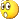# Orange pi win lvds support

Novice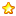credits
11Published in 2017-6-28 19:08:02 from mobile | Show all floors |Read mode
 There is support lvds up to 1366x768fps60 in datasheet of allwinner a64. But in documents it 3rd function on PD12-21. How to switch a display interface from LCD mipi dsi to lvds 1-ch 6-bit. I saw script where LCD dsi configuration is. So where I can get a conf of lvds. That's a most interesting thing in orange pi!

### This thread contains more resources

x

Novicecredits
11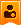Author| Published in 2017-6-30 21:53:01 | Show all floors
 so I found this: [disp_init] disp_init_enable = 1 disp_mode = 0 screen0_output_type = 1 screen0_output_mode = 4 screen1_output_type = 1 screen1_output_mode = 4 fb0_framebuffer_num = 2 fb0_format = 10 fb0_pixel_sequence = 0 fb0_scaler_mode_enable = 1 fb1_framebuffer_num = 2 fb1_format = 10 fb1_pixel_sequence = 0 fb1_scaler_mode_enable = 1 lcd0_bright = 197 lcd1_bright = 197 lcd0_screen_bright = 50 lcd0_screen_contrast = 50 lcd0_screen_saturation = 57 lcd0_screen_hue = 50 lcd1_screen_bright = 50 lcd1_screen_contrast = 50 lcd1_screen_saturation = 57 lcd1_screen_hue = 50 [lcd0_para] lcd_used = 1 lcd_x = 800 lcd_y = 480 lcd_dclk_freq = 30 lcd_pwm_not_used = 0 lcd_pwm_ch = 0 lcd_pwm_freq = 10000 lcd_pwm_pol = 0 lcd_if = 3 lcd_hbp = 200 lcd_ht = 1055 lcd_vbp = 25 lcd_vt = 1050 lcd_hv_if = 0 lcd_hv_smode = 0 lcd_hv_s888_if = 0 lcd_hv_syuv_if = 0 lcd_hv_vspw = 0 lcd_hv_hspw = 0 lcd_lvds_ch = 0 lcd_lvds_mode = 0 lcd_lvds_bitwidth = 0 lcd_lvds_io_cross = 0 lcd_cpu_if = 0 lcd_frm = 0 lcd_io_cfg0 = 268435456 lcd_gamma_correction_en = 0 lcd_gamma_tbl_0 = 0x0 lcd_gamma_tbl_1 = 0x10101 lcd_gamma_tbl_255 = 0xffffff lcd_bl_en_used = 0 lcd_bl_en = port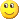H07<1><0><1> lcd_power_used = 1 lcd_power = portH08<1><0><1> lcd_pwm_used = 1 lcd_pwm = portB02<2><0> lcd_gpio_0 = lcd_gpio_1 = lcd_gpio_2 = lcd_gpio_3 = lcdd0 = portD00<2><0> lcdd1 = portD01<2><0> lcdd2 = portD02<2><0> lcdd3 = portD03<2><0> lcdd4 = portD04<2><0> lcdd5 = portD05<2><0> lcdd6 = portD06<2><0> lcdd7 = port:PD07<2><0> lcdd8 = port:PD08<2><0> lcdd9 = port:PD09<2><0> lcdd10 = port:PD10<2><0> lcdd11 = port:PD11<2><0> lcdd12 = port:PD12<2><0> lcdd13 = port:PD13<2><0> lcdd14 = port:PD14<2><0> lcdd15 = port:PD15<2><0> lcdd16 = port:PD16<2><0> lcdd17 = port:PD17<2><0> lcdd18 = port:PD18<2><0> lcdd19 = port:PD19<2><0> lcdd20 = port:PD20<2><0> lcdd21 = port:PD21<2><0> lcdd22 = port:PD22<2><0> lcdd23 = port:PD23<2><0> lcdclk = port:PH24<2><0> lcdde = port:PH25<2><0> lcdhsync = port:PH26<2><0> lcdvsync = port:PH27<2><0>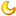Published in 2017-10-13 17:55:20 | Show all floors
 So... does it worked?